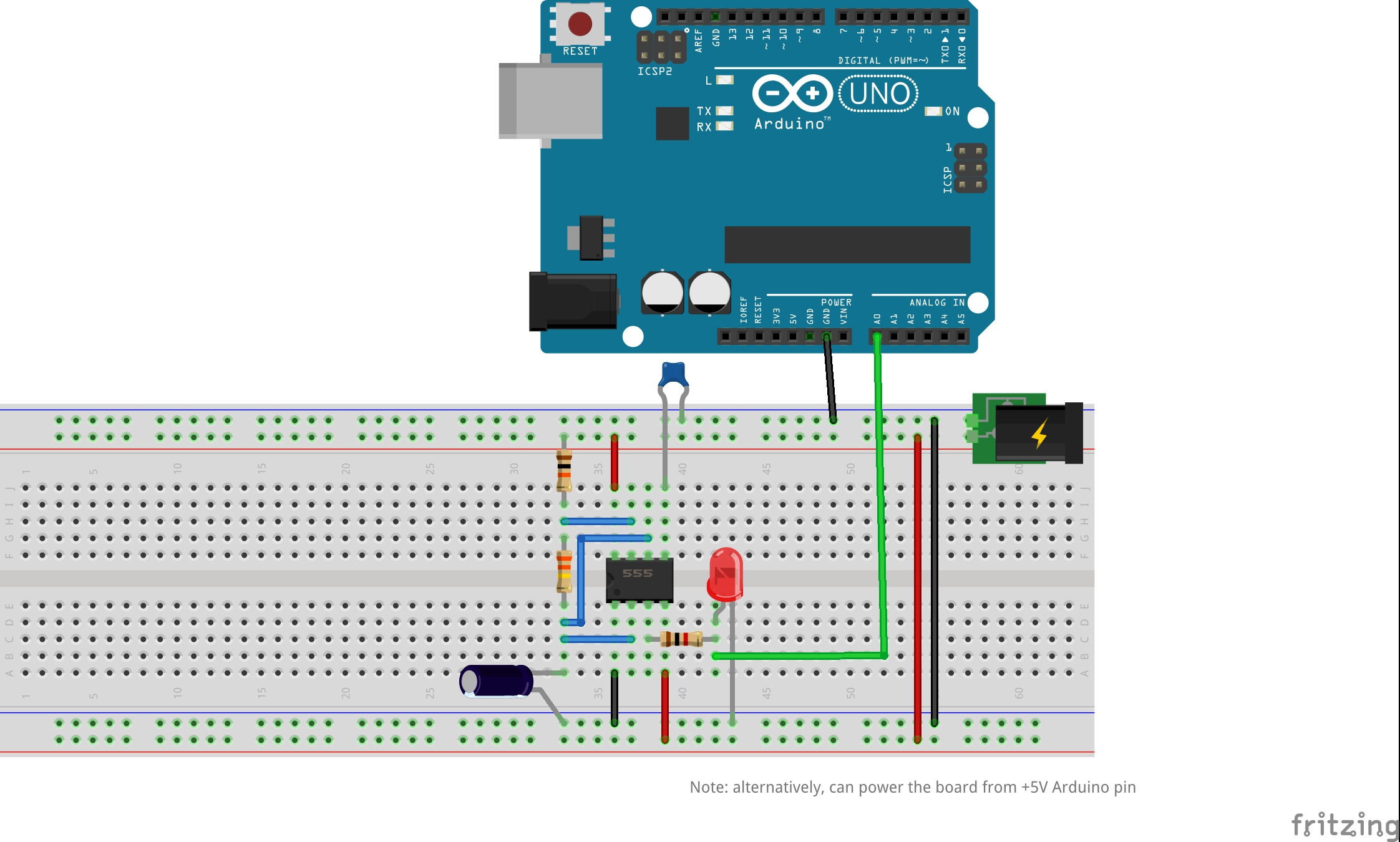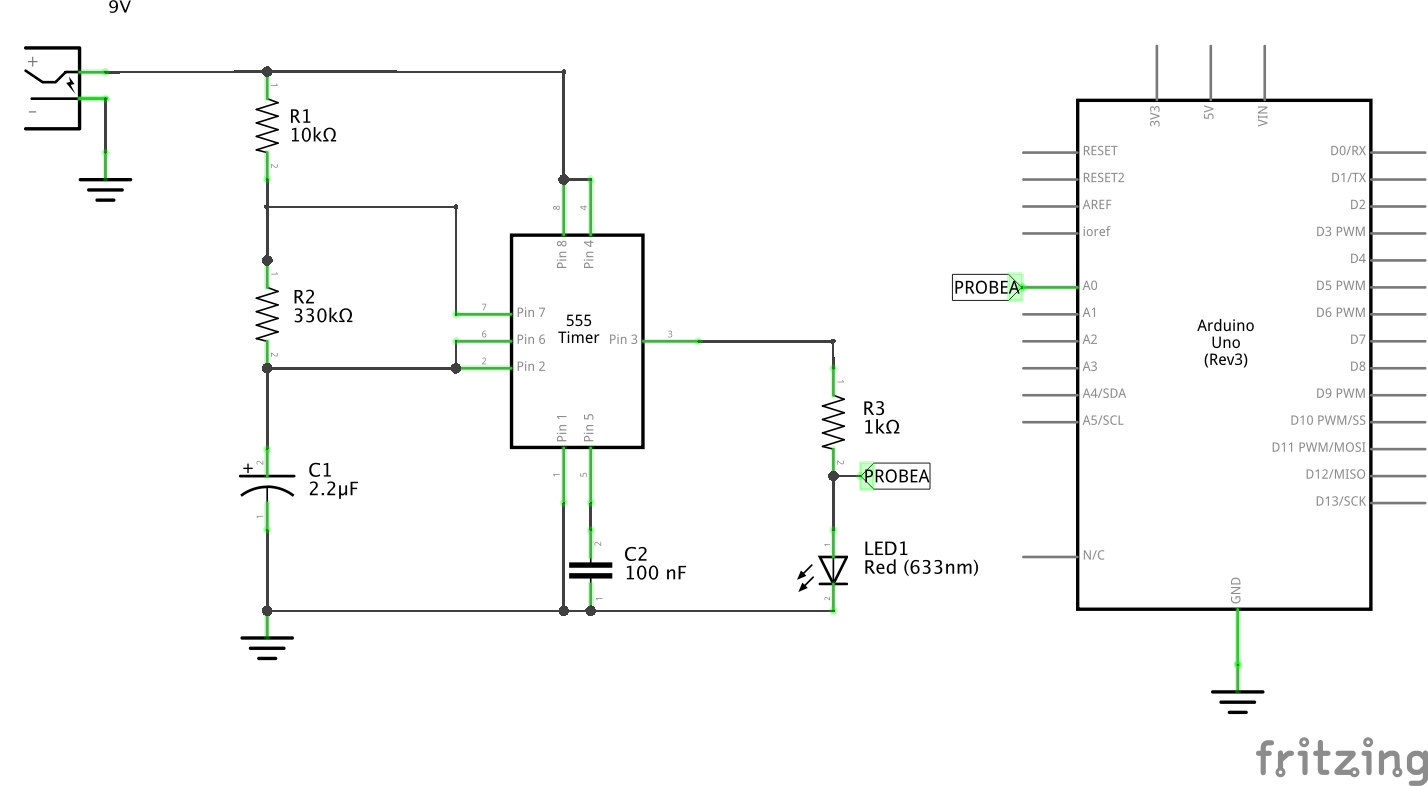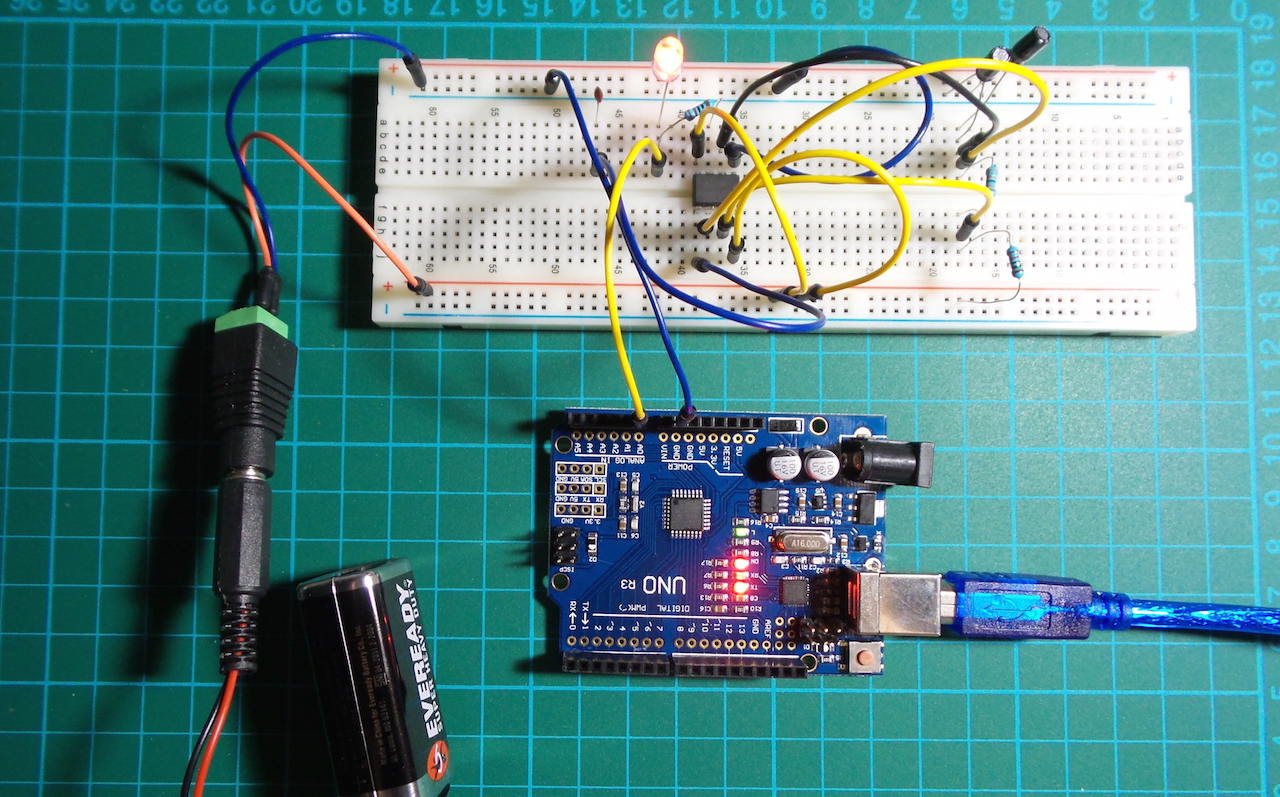# #016 555 Timer - A-Stable Oscillator

Use an Arduino to demonstrate the basic astable mode of operation, and plot the results with Processing.

Here’s a quick video of the circuit in action: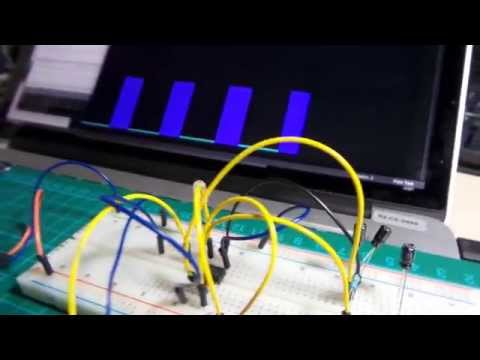The oscillator circuit is based on project 1 from Beginning Analog Electronics through Projects, and any number of other examples you can find with a quick Google search.

The a-stable mode of operation is actually well described in the LM555 Datasheet. This circuit produces a free-running square wave at the output pin 3, with frequency and duty cycle determined by the values of R1, R2, and C1.

### Selecting R1, R2, C1 Values

The circuit described uses R1=10k, R2=330k and C1=2.2uF, which results in a frequency of just about 1Hz and 50% duty cycle. i.e. half a second on, half a second off.

When selecting R1, R2, and C1 values, note that:

• the time low is a function of R2 and C1, so R1 will not affect it. Increasing R1 and C1 will increase the time low.
• the time high is a function of R1, 2*R2, C1. Increasing any of these values will increase the time high.
• the ratio of time high to time low is (R1 + R2)/R2, so the smaller R1 is compared to R2, the closer we get to a 50% duty cycle.
• the frequency is increased by reducing R1, R2 or C. But a change in R2 has twice the impact of R1 and C.

The formulae for calculating timings are:

``````t(high) = 0.693 x (R1 + R2) x C1
t(low) = 0.693 x R2 x C1
frequency = 1.44 / ((R1 + 2 x R2) x C1)
``````

See the datasheet for your chip to confirm the formulae. You can also find various websites that offer 555 timer calcuators, but I decided to write my own Visual 555 Calculator so that I could play around with HTML5 animation of the resulting circuit;-)

### Powering the project

The breadboard can be happily powered from an independent 9V supply, as indicated in the schematic. In this configuration, connecting the Arduino is purely optional – enabling the realtime processing plot of the output state.

However, it is also possible to power the board directly from the Arduino 5V pin.

### Output State Plots

The output voltage is tapped at the anode of the LED, read with an analog input pin and echoed to the Arduino serial port. It’s important to have the Arduino measure the voltage across the LED only, since with a 9V supply this will only be in the order of 2V or so, not the full 7V+ at the output pin 3 of the 555 timer.

PlotNValues (a simple Processing sketch) reads the data from the serial port and plots the output value over time, with some coloration effects thrown in for good measure.

Here’s a sample trace for R1=10k, R2=330k and C1=2uF: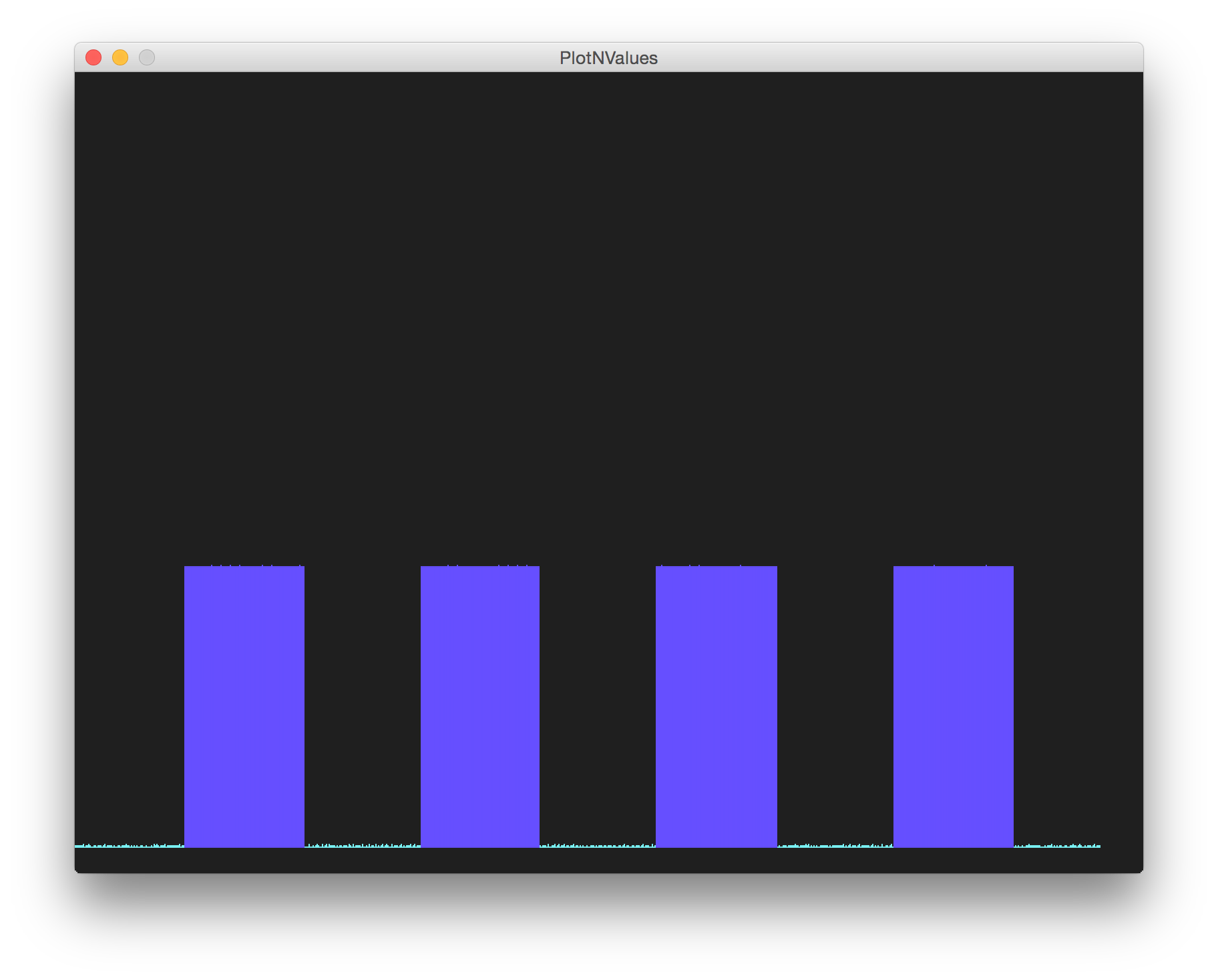### Construction